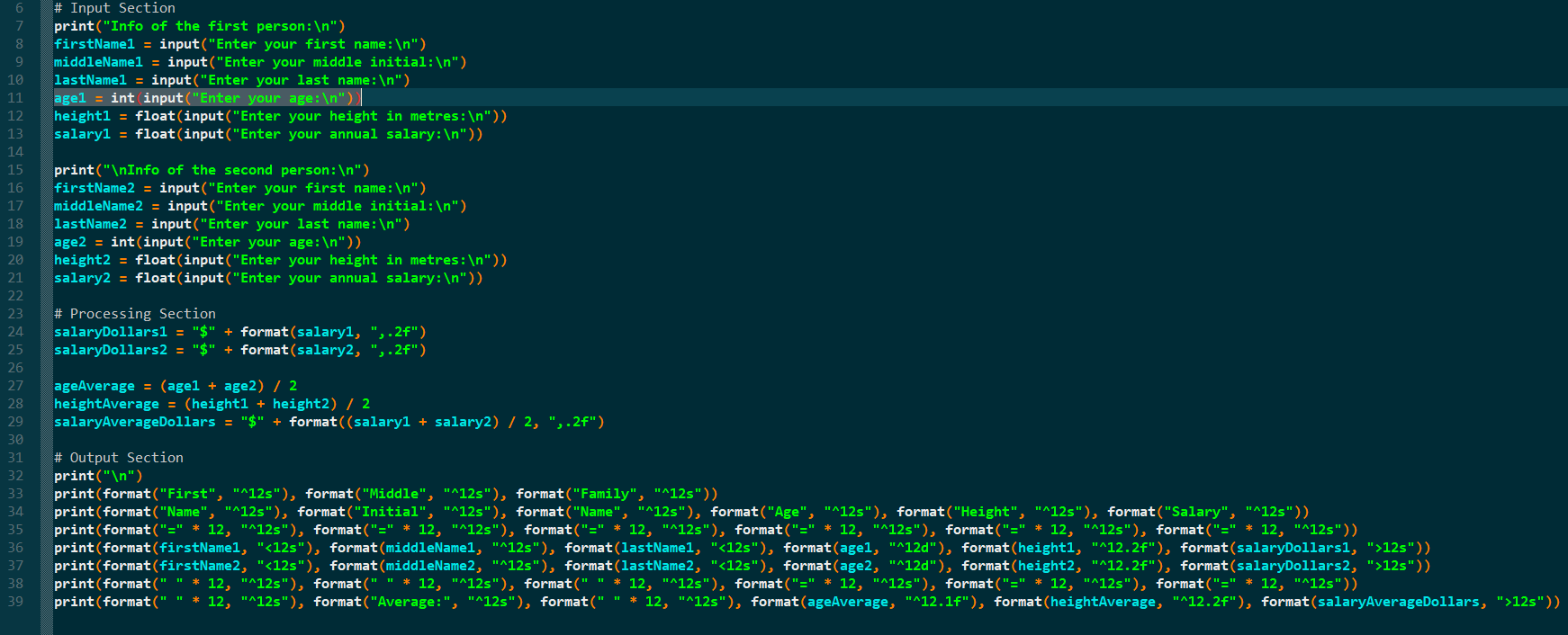Ask coding questions

← Back to all posts
##### ColinKirschAssignment#2d.py

this is supposed to be a program that finds the average age, height, and salary of two people and changes the salary to \$. i cant get the invalid syntax thing gone so im posting this for you guys to tell me what i did wrong and how to fix it. like how to get the syntax gone. please help me.

##### Comments
hotnewtop
ColinKirsch (0)

your thing doesnt work. it is suposed to look like the link im putting here. all the code i had was suposed to be there but the syntax will not go away

IcynDevz (764)

@ColinKirsch but what does all this do

``````print('\n')
print(format('First', '^12s'), format('Middle', '^12s'), format('Family', '^12s'))
print(format('Name', '^12s'), format('Initial', '^12s'), format('Name', '^12s'), format('Age', '^12s'), format('Height', '^12s'), (format('Salary', '^12s'))
print(format('='*12, '^12s'), format('='*12, '^12s'), format('='*12, '^12s'), format('='*12, '^12s'), format('='*12, '^12s'), format('='*12, '^12s'))
print(format(firstName1, '<12s'), format(middleName1, '^12s'), format(familyName1, '<12s'), format(age1, '^12d'), format(height1, '^12.2f'), format(salary1, '>12s'))
print(format(firstName2, '<12s'), format(middleName2, '^12s'), format(familyName2, '<12s'), format(age2, '^12d'), format(height2, '^12.2f'), format(salary2, '>12s'))
print(format(' '*12, '^12s'), format(' '*12, '^12s'), format(' '*12, '^12s'), format('='*12, '^12s'), format('='*12, '^12s'), format('='*12, '^12s'), format('='*12, '^12s'))
print(format(''*12, "^12s"), format('Average:' *12, '^12s'), format(''*12, '^12s'), format(ageAverage,*12, '^12s'), format(heightAverage*12, '^12s'), format(salaryAverageDollars*12, '>12s'))``````
ColinKirsch (0)

the assignment was to make a chart like the one i have in my last comment. basicly the code is suposed to look like thisso i an trying to do this before 5 pm when it is due.
@IcingHackz